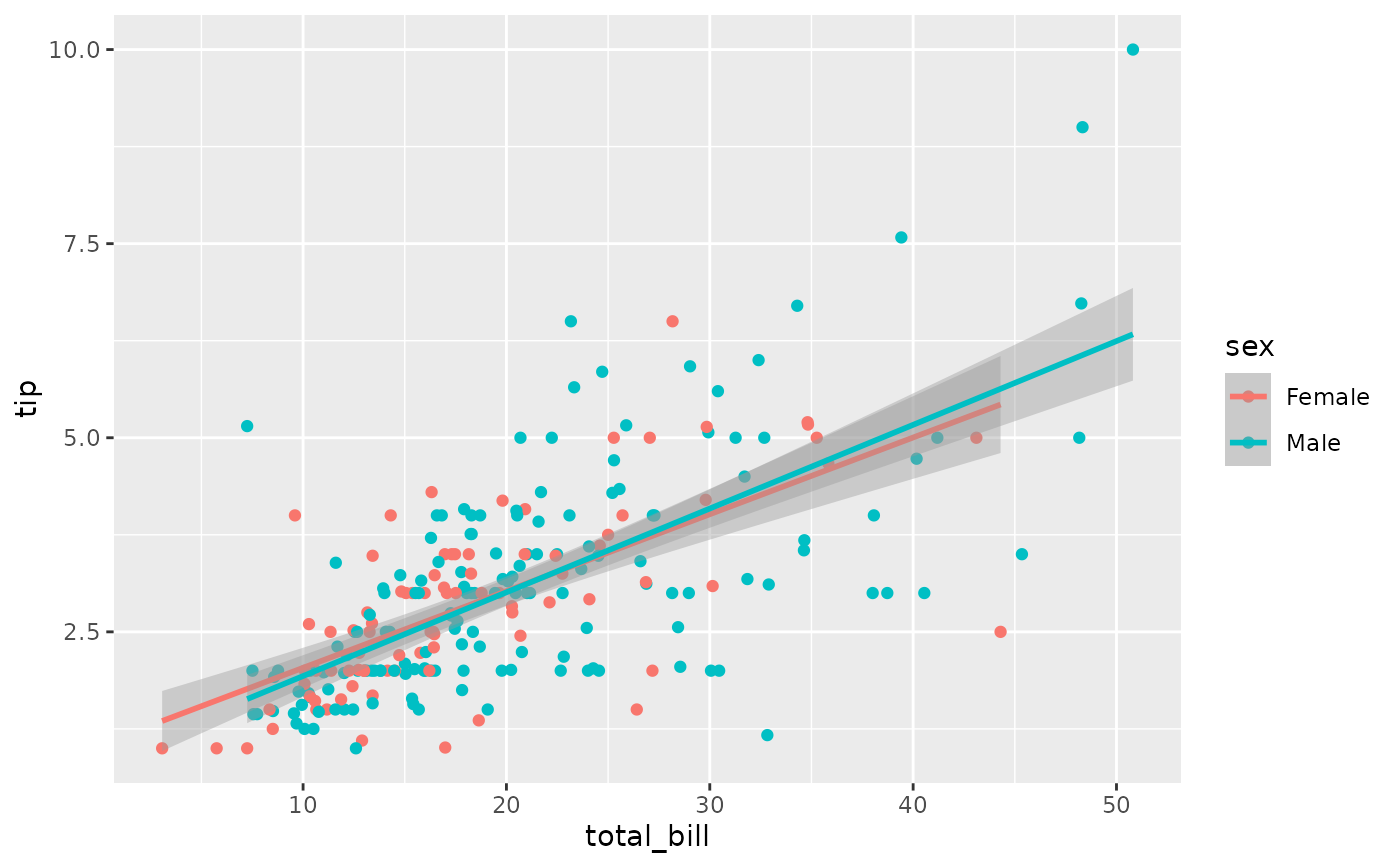Add a smoothed condition mean with a given scatter plot.

ggally_smooth(
data,
mapping,
...,
method = "lm",
formula = y ~ x,
se = TRUE,
shrink = TRUE
)

ggally_smooth_loess(data, mapping, ...)

ggally_smooth_lm(data, mapping, ...)

## Arguments

data data set using aesthetics being used parameters supplied to geom_smooth other arguments to add to geom_smooth boolean to determine if y range is reduced to range of points or points and error ribbon

## Details

Y limits are reduced to match original Y range with the goal of keeping the Y axis the same across plots.

Barret Schloerke

## Examples

# Small function to display plots only if it's interactive
p_ <- GGally::print_if_interactive

data(tips, package = "reshape")
p_(ggally_smooth(tips, mapping = ggplot2::aes(x = total_bill, y = tip)))p_(ggally_smooth(tips, mapping = ggplot2::aes_string(x = "total_bill", y = "tip")))p_(ggally_smooth(tips, mapping = ggplot2::aes_string(x = "total_bill", y = "tip", color = "sex")))